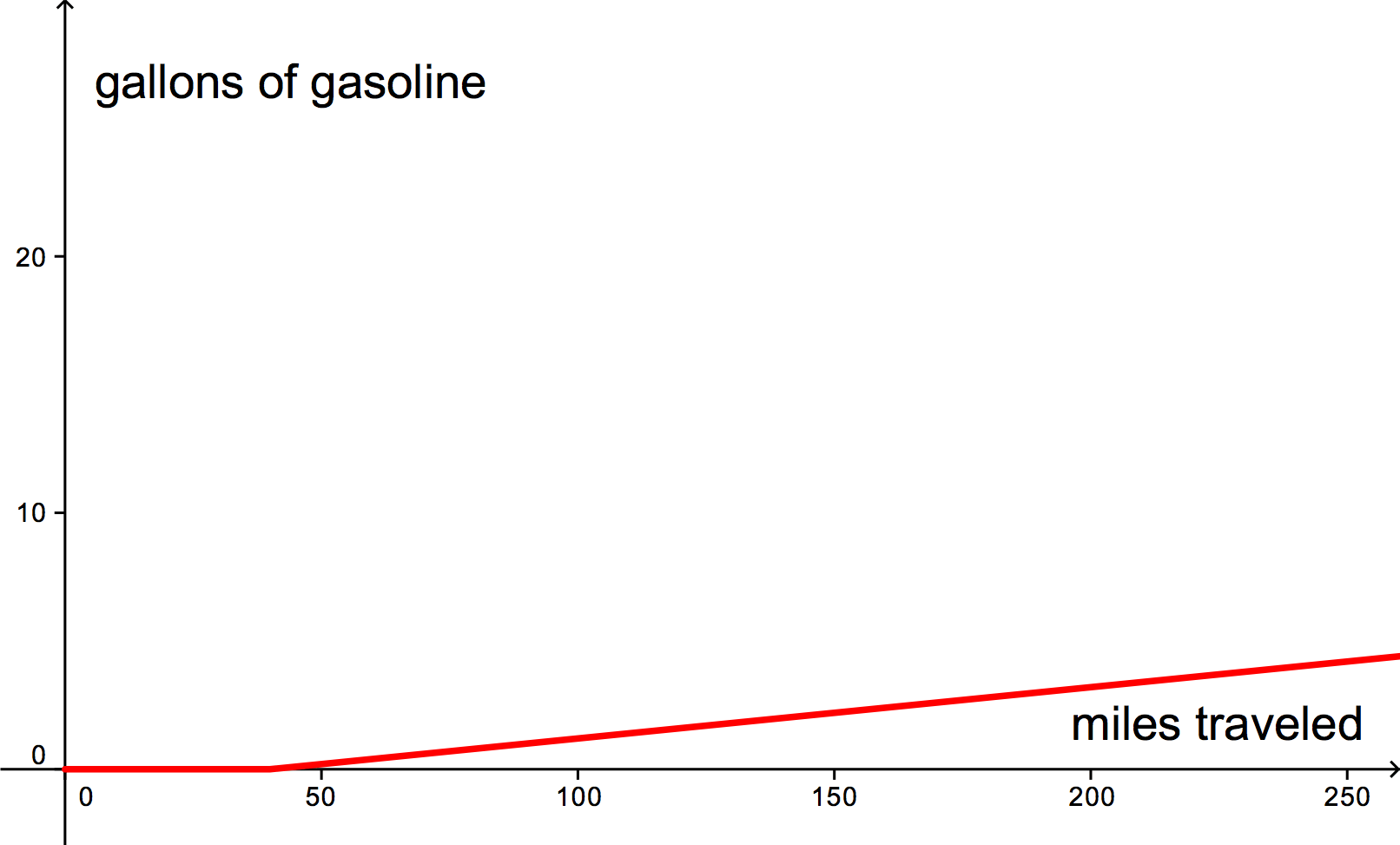# Calculating miles per gallon for electric cars

GM says new Volt could get 230 mpg in city driving.” This was a headline yesterday about GM’s new electric car. Wow, pretty impressive!

But wait,… what?!? This car (which seems quite promising, actually) has batteries that can propel the car for approximately 40 miles. Only after the batteries are empty does a gas-powered generator kick on to recharge the batteries. Does miles per gallon have any meaning in this context?

The problem is that fuel consumption as a function of miles traveled is not linear. In fact it is a piecewise function consisting of a linear part (always zero) and an affine part. According to the article, when the gas generator is running, the car gets approximately 50 mpg. So, if we have fully-charged batteries, the amount of gasoline consumed after$m$ miles traveled is$\displaystyle f(m)= \left\{\begin{array}{lr}0 & m \le 40\\ (m-40)/50 & m>40.\end{array}\right.$How do we compute miles per gallon for such a car? Here’s one idea. If we start a trip with a fully charged battery and drive$m$ miles, then we could divide$m$ by the number of gallons of gas consumed. This would give our average miles per gallon for the trip.

We can express this more formally as the function$a(m)=m/f(m)$. Interestingly this function is “infinite” for the first 40 miles. After that$a(m)=m/f(m)=50m/(m-40)$. Thus, not surprisingly, the number of miles per gallon approaches 50 as$m$ goes to infinity.Notice that we hit 230 mpg at slightly over 51 miles. Thus if you drove fewer than 51 miles each day and fully recharged the batteries each night, then you would do better than the advertised 230 miles per gallon.

Of course, all of this is silly. Miles per gallon is a useful measure if that is the only fuel that you are putting into your car, because then it gives you an indication of how much it would cost you to drive. But electricity is not free. Probably a better measure would be cents per mile, but of course this would fluctuate as the prices of both gasoline and electricity go up and down and it would depend on your driving habits—not so easy to put on a window sticker. The article gives the following information regarding the cost for charging.

Henderson said charging the Volt will cost about 40 cents a day, at about 5 cents per kilowatt hour.

Unfortunately, this quote gives too little information to be useful. It would be nice to know how much it would cost to fully recharge empty batteries (I can’t imagine that that is what this 40 cent figure is referring to).

According to the article, the EPA is still trying to figure out how properly quantify fuel efficiency:

The EPA is working with the Society of Automotive Engineers and state and federal officials to develop testing procedures to measure the fuel efficiency of advanced vehicles, according to a draft outline of the proposal obtained by The Associated Press.

I should mention that another incentive for getting a car like this is that it decreases our dependence on oil, which is an admirable goal. But switching to fully electric cars just moves the debate over to another field of energy—where does the electricity come from, what effect will this have on our power grid, etc.? Good questions, but not the point of this post.